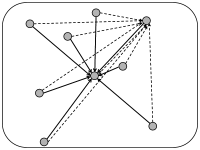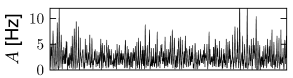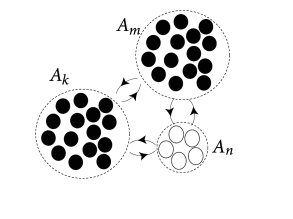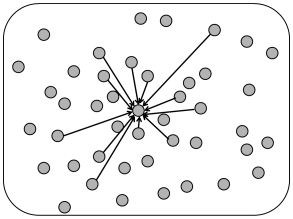# 12.3 Connectivity Schemes

The real connectivity between cortical neurons of different types and different layers, or within groups of neurons of the same type and the same layer is still partially unknown, because experimental data is limited. At most, some plausible estimates of connection probabilities exist. In some cases the connection probability is considered as distance-dependent, in other experimental estimates as uniform in the restricted neighborhood of a cortical column.

In simulations of spiking neurons, there are a few coupling schemes that are frequently adopted (Fig. 12.6). Most of these assume random connectivity within and between populations. In the following we discuss these schemes with a special focus on the scaling behavior induced by each choice of coupling scheme. Here, scaling behavior refers to a change in the number $N$ of neurons that participate in the population.

An understanding of the scaling behavior is important not only for simulations, but also for the mathematical analysis of the network behavior. However, it should be kept in mind that real populations of neurons have a fixed size because, e.g., the number of neurons in a given cortical column is given and, at least in adulthood, does not change dramatically from one day to the next. Typical numbers, counted in one column of mouse somatosensory cortex (barrel cortex, C2) are 5750 excitatory and 750 inhibitory neurons (293). Thus numbers are finite and considerations of the behavior for the number $N$ going to infinity are of little relevance to biology.

We note that numbers are even smaller if we break them down per layer. The estimated mean ($\pm$ standard error of the mean) number of excitatory neurons in each layer (L1 to L6) are as follows: L2, 546 $\pm$ 49; L3, 1145 $\pm$ 132; L4, 1656 $\pm$ 83; L5A, 454 $\pm$ 46; L5B, 641 $\pm$ 50; L6, 1288 $\pm$ 84 and for inhibitory neurons: L1, 26 $\pm$ 8; L2, 107 $\pm$ 7; L3, 123 $\pm$ 19; L4, 140 $\pm$ 9; L5A, 90 $\pm$ 14; L5B, 131 $\pm$ 6; L6, 127 $\pm$ 9 (293).Fig. 12.6: Coupling Schemes. A. Full connectivity: Top: A network of 9 neurons with all-to-all coupling. The input links are shown for two representative neurons. Self-couplings are not indicated. Bottom: The number of input links (indicated for one representative neuron) increases, if the size of the network is doubled. B. Random coupling with fixed connection probability. In a network of 18 neurons (bottom) the number of input links is larger than in a network of 9 neurons (top). C. Random coupling with fixed number of inputs. The number of links from presynaptic neurons (top: input links to two representative neurons) does not change when the size of the network is increased (bottom: input links to one representative neuron).

Example: Scaling of interaction strength in networks of different sizes

Suppose we simulate a fully connected network of 1 000 noisy spiking neurons. Spikes of each neuron in the population generate in all other neurons an inhibitory input current of strength $w<0$ which lasts for 20 milliseconds. In addition to the inhibitory inputs, each neuron also receives a fixed constant current $I_{0}^{\rm ext}$ so that each neurons in the network fires at 5Hz. Since each neuron receives input from itself and from 999 partner neurons, the total rate of inhibitory input is 5kHz. Because each input exerts an effect over 20 milliseconds, a neuron is, at each moment in time, under the influence of about 100 inhibitory inputs - generating a total input $I\approx 100\,w+I_{0}^{\rm ext}$.

We now get access to a bigger computer which enables us to simulate a network of 2 000 neurons instead of 1 000. In the new network each neuron therefore receives inhibitory inputs at a rate of 10kHz and is, at each moment in time, under the influence of a total input current $I\approx 200\,w+I_{0}^{\rm ext}$. Scaling the synaptic weights $w$ by a factor of 1/2 leads us back to the same total input, as before.

Why should we be interested in changing the size of the network? As mentioned before, in biology the network size is fixed. An experimentalist might tell us that the system he studies contains 20 000 neurons, connected with each other with strength $w_{ij}=1$ (in some arbitrary units) and connection probability of 10%. Running a simulation of the full network of 20 000 neurons is possible, but will take a certain amount of time. We may want to speed up the simulation by simulating a network of 4 000 neurons instead. The question arises whether we should increase the interaction strength in the smaller network compared to the value $w_{ij}=1$ in the big network.

# 12.3.1 Full connectivity

The simplest coupling scheme is all-to-all connectivity within a population. All connections have the same strength. If we want to change the number $N$ of neurons in the simulation of a population, an appropriate scaling law is

 $w_{ij}={J_{0}\over N}\,.$ (12.6)

This scaling law is a mathematical abstraction that enables us to formally take the limit of $N\to\infty$ while keeping the expected input that a neuron receives from its partners in the population fixed. In the limit of $N\to\infty$ limit, the fluctuations disappear and the expected input can be considered as the actual input to any of the $N$ neurons. Of course, real populations are of finite size, so that some fluctuations always remain. But as $N$ increases the fluctuations decrease.

A slightly more intricate all-to-all coupling scheme is the following: weights $w_{ij}$ are drawn from a Gaussian distribution with mean $J_{0}/N$ and standard deviation $\sigma_{0}/\sqrt{N}$. In this case, each neuron in the population sees a somewhat different input, so that fluctuations of the membrane potential are of the order $\sigma_{0}$ even in the limit of large $N$ (146).Fig. 12.7: Simulation of a model network with a fixed connection probability p=0.1p=0.1. A. Top: Population activity A⁢(t)A(t) averaged over all neurons in a network of 4 000 excitatory and 1 000 inhibitory neurons. Bottom: Total input current Ii⁢(t)I_{i}(t) into two randomly chosen neurons. B. Same as A, but for a network with 8 000 excitatory and 2 000 inhibitory neurons. The synaptic weights have been rescaled with a factor of 1/21/2 and a common input current IextI^{\rm ext} is given to all neurons to ensure that the same population activity is obtained. All neurons are leaky integrate-and-fire units with identical parameters interacting by short current pulses.

# 12.3.2 Random coupling: Fixed coupling probability

Experimentally the probability $p$ that a neuron inside a cortical column makes a functional connection to another neuron in the same column is in the range of 10 percent, but varies across layers; cf. Fig. 12.5.

In simulations, we can fix a connection probability $p$ and choose connections randomly with probability $p$ among all the possible $N^{2}$ connections. In this case, the number of presynaptic input links $C_{j}$ to a postsynaptic neuron $j$ has a mean value of $\langle C_{j}\rangle=pN$, but fluctuates between one neuron and the next with variance $p(1-p)N$.

Alternatively, we can take one model neuron $j=1,2,3,\dots N$ after the other and choose randomly $C=pN$ presynaptic partners for it (each neuron can be picked only once as a presynaptic partner for a given postsynaptic neuron $j$). In this case all neurons have, by construction, the same number of input links $C_{j}=C$. By an analogous selection scheme, we could also fix the number of output links to exactly $p\,N$ as opposed of simply imposing $p\,N$ as the average value.

Whatever the exact scheme to construct random connectivity, the number of input connections per neuron increases linearly with the size $N$ of the population; see Fig. 12.6B. It is therefore useful to scale the strength of the connections as

 $w_{ij}={J_{0}\over C}={J_{0}\over p\,N}\,,$ (12.7)

so that the mean input to typical neuron does not change if the number of model neurons in the simulated population is increased. Since in a bigger network individual inputs have less effects with the scaling according to Eq. (12.7), the amount of fluctuations in the input decreases with population size; compare Fig. 12.7.

# 12.3.3 Random coupling: Fixed number of presynaptic partners

The number of synapses onto the dendrites of a single pyramidal neuron is estimated to lie in the range of a few thousand (249). Thus, when one simulates networks of a hundred thousand neurons or millions of neurons, a modeling approach based on a fixed connection probability in the range of 10 percent cannot be correct. Moreover, in an animal participating in an experiment, not all neurons will be active at the same time. Rather only a few subgroups will be active, the composition of which depends on the stimulation conditions and the task. In other words, the number of inputs converging onto a single neuron may be much smaller than a thousand.

We can construct a random network with a fixed number $C$ of inputs by the following procedure. We pick one model neuron $j=1,2,3,\dots N$ after the other and choose randomly its $C$ presynaptic partners; see Fig. 12.6C. Whenever the network size $N$ is much bigger than $C$, the inputs to a given neuron can be thought of as random samples from the current network activity. No scaling of the connections with the population size $N$ is necessary; see Fig. 12.8.Fig. 12.8: Simulation of a model network with a fixed number of presynaptic partners (400 excitatory and 100 inhibitory cells) for each postsynaptic neuron. A. Top: Population activity A⁢(t)A(t) averaged over all neurons in a network of 4 000 excitatory and 1 000 inhibitory neurons. Bottom: Total input current Ii⁢(t)I_{i}(t) into two randomly chosen neurons. B. Same as A, but for a network with 8 000 excitatory and 2 000 inhibitory neurons. The synaptic weights have not been rescaled. While the fluctuations of the population activity A⁢(t)A(t) decrease compared to the smaller network (top), the mean and variance of the synaptic input do not change with the size of the network (bottom). All neurons are leaky integrate-and-fire units with identical parameters interacting by current pulses; cf. (79).

# 12.3.4 Balanced excitation and inhibition

In the simulations of Fig. 12.2, we have assumed a network of excitatory and inhibitory neurons. In other words, our network consists of two interacting populations. The combination of one excitatory and one inhibitory population can be exploited for the scaling of synaptic weights, if the effects of excitation and inhibition are ’balanced’.

In the discussion of scaling in the previous paragraphs, it was mentioned that a fixed connection probability of $p$ and a scaling of weights $w_{ij}=J_{0}/(p\,N)$ leads to a mean neuronal input current that is insensitive to the size of the simulation; however, fluctuations decrease with increasing $N$. Is there a possibility to work with a fixed connection probability $p$ and yet control the size of the fluctuations while changing $N$?

In a network of two populations, one excitatory and one inhibitory, it is possible to adjust parameters such that the mean input current into a typical neuron vanishes. The condition is that the total amount of excitation and inhibition cancel each other, so that excitation and inhibition are ‘balanced’. The resulting network is called a balanced network or a population with balanced excitation and inhibition (532; 539).

If the network has balanced excitation and inhibition the mean input current to a typical neuron is automatically zero and we do not have to apply a weight rescaling scheme to control the mean. Instead, we can scale synaptic weights so as to control specifically the amount of fluctuations of the input current around zero. An appropriate choice is

 $w_{ij}={J_{0}\over\sqrt{C}}={J_{0}\over\sqrt{pN}}\,.$ (12.8)

With this choice, a change in the size of the network hardly affects the mean and variance of the input current into a typical neuron; cf. Fig. 12.9. Note that in simulations of networks of integrate-and-fire neurons, the mean input current to the model neurons is in practice often controlled, and adjusted, through a common constant input to all neurons. In Figs. 12.7 - 12.9 we simply report the main effects of network size on the population activity and synaptic currents; the analysis of the observed phenomena will be postponed to Section 12.4.Fig. 12.9: Simulation of a model network with balanced excitation and inhibition and fixed connectivity p=0.1p=0.1 A. Top: Population activity A⁢(t)A(t) averaged over all neurons in a network of 4 000 excitatory and 1 000 inhibitory neurons. Bottom: Total input current Ii⁢(t)I_{i}(t) into two randomly chosen neurons. B. Same as A, but for a network with 8 000 excitatory and 2 000 inhibitory neurons. The synaptic weights have been rescaled by a factor 1/21/\sqrt{2} and the common constant input has been adjusted. All neurons are leaky integrate-and-fire units with identical parameters coupled interacting by short current pulses.

# 12.3.5 Interacting Populations

In the previous paragraph we already considered two populations, one of them excitatory and the other one inhibitory. Let us generalize the arguments to a network consisting of several populations; cf. Fig. 12.10. It is convenient to visualize the neurons as being arranged in spatially separate pools, but this is not necessary. All neurons could, for example, be physically localized in the same column of the visual cortex, but of three different types: excitatory, fastspiking inhibitory, and non-fastspiking interneurons.Fig. 12.10: Several interacting populations of neurons. A. A neuron in population Γk\Gamma_{k} is driven by the population activity AmA_{m} and AnA_{n} of other groups, as well as by the activity AkA_{k} of its own population. B. All neurons in group Γn\Gamma_{n} are coupled with synaptic efficacy wi⁢j=Jn⁢n/Nnw_{ij}=J_{nn}/N_{n}. Each pair of neurons i,ji,j with the presynaptic jj in groups Γm\Gamma_{m} and the postsynaptic neuron ii in Γn\Gamma_{n} is coupled via wi⁢j=Jn⁢m/Nmw_{ij}=J_{nm}/N_{m}.

We assume that neurons are homogeneous within each pool. The activity of neurons in pool $n$ is

 $A_{n}(t)={1\over N_{n}}\sum_{j\in\Gamma_{n}}\sum_{f}\delta(t-t_{j}^{(f)})\,,$ (12.9)

where $N_{n}$ is the number of neurons in pool $n$ and $\Gamma_{n}$ denotes the set of neurons that belong to pool $n$. We assume that each neuron $i$ in pool $n$ receives input from all neurons $j$ in pool $m$ with strength $w_{ij}=J_{nm}/N_{m}$; cf. Fig. 12.10. The time course $\alpha_{ij}(s)$ caused by a spike of a presynaptic neuron $j$ may depend on the synapse type, i.e., a connection from a neuron in pool $m$ to a neuron in pool $n$, but not on the identity of the two neurons. The input current to a neuron $i$ in group $\Gamma_{n}$ is generated by the spikes of all neurons in the network,

 $\displaystyle I_{i,n}$ $\displaystyle=$ $\displaystyle\sum_{j}\sum_{f}w_{ij}\,\alpha_{ij}(t-t_{j}^{(f)})$ (12.10) $\displaystyle=$ $\displaystyle\sum_{m}J_{nm}\int_{0}^{\infty}\alpha_{nm}(s)\,\sum_{j\in\Gamma_{% m}}\sum_{f}{\delta(t-t_{j}^{(f)}-s)\over N_{m}}ds\,,$

where $\alpha_{nm}(t-t_{j}^{(f)})$ denotes the time course of a postsynaptic current caused by spike firing at time $t_{j}^{(f)}$ of the presynaptic neuron $j$ which is part of population $m$. We use Eq. (12.9) to replace the sum on the right-hand side of Eq. (12.10) and obtain

 $I_{n}=\sum_{m}J_{nm}\int_{0}^{\infty}\alpha(s)\,A_{m}(t-s)\,{\text{d}}s\,.$ (12.11)

We have dropped the index $i$ since the input current is the same for all neurons in pool $n$.

Thus, we conclude that the interaction between neurons of different pools can be summarized by the population activity $A(t)$ of the respective pools. Note that Eq. (12.11) is a straightforward generalization of Eq. (12.4) and could have been ‘guessed’ immediately; external input $I^{\rm ext}$ could be added as in Eq. (12.4).

# 12.3.6 Distance dependent connectivity

The cortex is a rather thin sheet of cells. Cortical columns extend vertically across the sheet. As we have seen before, the connection probability within a column depends on the layer where pre- and postsynaptic neurons are located. In addition to this vertical connectivity, neurons make many horizontal connections to neurons in other cortical columns in the same, but also in other areas of the brain. Within the same brain area the probability of making a connection is often modeled as distance dependent. Note that distance dependence is a rather coarse feature, because the actual connectivity depends also on the function of the pre- and postsynaptic cell. In the primary visual area, for example, it has been found that pyramidal neurons with a preferred orientation for horizontal bars are more likely to make connections to other columns with a similar preferred orientation (27).

For models of distance-dependent connectivity it is necessary to assign to each model neuron $i$ a location $x(i)$ on the two-dimensional cortical sheet. Two different algorithmic procedures can be used to assign distance-dependent connectivity (Fig. 12.11). The first one assumes full connectivity with a strength $w_{ij}$ which falls off with distance

 $w_{ij}=w(|x(i)-x(j)|),$ (12.12)

where $x(i)$ and $x(j)$ denote the location of post- and presynaptic neurons, respectively, and $w$ is a function into the real numbers. For convenience, one may assume finite support so that $w$ vanishes for distances $|x(i)-x(j)|>d$.

The second alternative is to give all connections the same weight, but to assume that the probability $P$ of forming a connection depends on the distance

 ${\rm Prob}(w_{ij}=1)=P(|x(i)-x(j)|)\,,$ (12.13)

where $x(i)$ and $x(j)$ denote the location of post- and presynaptic neurons, respectively and $P$ is a function into the interval $[0,1]$.Fig. 12.11: Distance dependent connectivity. A. Random coupling where the probability of making a connection falls off with distance. Incoming connections to a single selected neuron are shown. B. Full connectivity in a local neighborhood, but the connection strength falls off as a function of distance. Incoming connections to a single selected neuron are strong (thick solid arrow among close neighbors), weak (dashed) or non-existent (for distant neurons).

Example: Expected number of connections

Let us assume that the density of cortical neurons at location $y$ is $\rho(y)$. The expected number of connection that a single neuron $i$ located at position $x(i)$ receives is then $C_{i}=\int P(|x(i)-y|)\,\rho(y)\,dy$. If the density is constant, $\rho(y)=\rho_{0}$, then the expected number $C$ of input synapses is the same for all neurons and controlled by the integral of the connection probability, i.e., $C_{i}=\rho_{0}\,\int P(|x(i)-y|)\,dy$.

# 12.3.7 Spatial Continuum Limit (*)

The physical location of a neuron in a population often reflects the task of a neuron. In the auditory system, for example, neurons are organized along an axis that reflects the neurons’ preferred frequency. A neuron at one end of the axis will respond maximally to low-frequency tones; a neuron at the other end to high frequencies. As we move along the axis the preferred frequency changes gradually. In the visual cortex, the preferred orientation changes gradually as one moves along the cortical sheet. For neurons organized in a spatially extended multidimensional network, a description by discrete pools does not seem appropriate. We will indicate in this section that a transition from discrete pools to a continuous population is possible. Here we give a short heuristic motivation of the equations. A thorough derivation along a slightly different line of arguments will be performed in Chapter 18.

To keep the notation simple, we consider a population of neurons that extends along a one-dimensional axis; cf. Fig. 12.12. We assume that the interaction between a pair of neurons $i,j$ depends only on their location $x$ or $y$ on the line. If the location of the presynaptic neuron is $y$ and that of the postsynaptic neuron is $x$, then $w_{ij}=w(x,y)$. In other words we assume full, but spatially dependent connectivity and neglect potential random components in the connectivity pattern.

In order to use the notion of population activity as defined in Eq. (12.11), we start by discretizing space in segments of size $d$. The number of neurons in the interval $[n\,d,(n+1)\,d]$ is $N_{n}=\rho\,d$ where $\rho$ is the spatial density. Neurons in that interval form the group $\Gamma_{n}$.Fig. 12.12: In a spatially continuous ensemble of neurons, the number of neurons in a segment dd is N=ρ⁢dN=\rho\,d. The efficacy wi⁢jw_{ij} between two neurons depends on their location. The coupling strength between a presynaptic neuron jj at position x⁢(j)≈m⁢dx(j)\approx md and a postsynaptic neuron ii at location x⁢(i)≈n⁢dx(i)\approx nd is wi⁢j≈w⁢(n⁢d,m⁢d)w_{ij}\approx w(nd,md).

We now change our notation with respect to the discrete population and replace the subscript $m$ in the population activity $A_{m}$ by the spatial position of the neurons in that group

 $\displaystyle A_{m}(t)$ $\displaystyle\longrightarrow$ $\displaystyle A(md,t)=A(y,t)\,.$ (12.14)

Since the efficacy of a pair of neurons with $i\in\Gamma_{n}$ and $j\in\Gamma_{m}$ is by definition $w_{ij}=J_{nm}/N_{m}$ with $N_{m}=\rho\,d$, we have $J_{nm}=\rho\,d\,w(nd,md)$. We use this in Eq. (12.11) and find for the input current

 $I(nd,t)=\rho\sum_{m}d\,w(nd,md)\int_{0}^{\infty}\alpha(s)\,A(md,t-s)\,{\text{d% }}s\,,$ (12.15)

where $\alpha(s)$ describes the time course of the postsynaptic current caused by spike firing in one of the presynaptic neurons. For $d\to 0$, the summation on the right-hand side can be replaced by an integral and we arrive at

 $I(x,t)=\rho\int w(x,y)\int_{0}^{\infty}\alpha(s)\,A(y,t-s)\,{\text{d}}s\,{% \text{d}}y\,,$ (12.16)

which is the final result. To rephrase Eq. (12.16) in words, the input to neurons at location $x$ depends on the spatial distribution of the population activity convolved with the spatial coupling filter $w(x,y)$ and the temporal filter $\alpha(s)$. The population activity $A(y,t-s)\Delta s$ is the number of spikes in a short interval $\Delta s$ summed across neurons in the neighborhood around $y$ normalized by the number of neurons in that neighborhood.